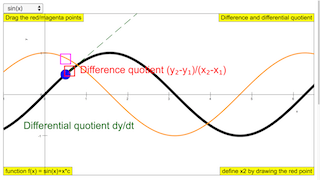Intro Page

# Difference quotient/ differential  quotient

The transition from the difference quotient to the differential quotient (first derivative) is demonstrated for the sine function.

y = sin(x)

Difference quotient (x1 ) = (y2 - y1)/(x2 -x1)

Differential quotient dy/dt (x1 ) = Limit for x2→ x1 of (y2 - y1)/(x2 -x1)

The zero ordinate line y=0 is drawn in red. The blue model point x1 , y1, is defined with the slider. Drawing the red point with the mouse defines the second point x2 , y2. The line connecting both points (secant) is drawn in black, and is prolongated beyond the second point in green.

The difference of the ordinates (y2 - y1) is shown as a red arrow, the difference of the abscissa (x2 -x1) as a blue one.

The difference quotient (y2 - y1)/(x2 -x1), which is the tangent of the angle between both components at the model point, is shown as a magenta colored point.

The analytic first derivative function cos(x) = d(sin(x))/dx is drawn as a beige line.

Choose any arbitrary model point with the slider. Draw the red point closer and closer to the blue model point. In the limit of this process the secant turns into a tangent at the model point, and the difference quotient becomes a point on the derivative = the differential quotient at the model point.

1st and 2nd

# First and second derivative

The simulation demonstrates the limit process in deriving the first derivative of a sine function with a superimposed linear term (a straight line) . For this case the second derivative should be independent of the linear term.

y = sin(x) +c x

dy/dt(x1) = x2➙x1 lim (y2 - y1 )/(x2 - x1) = cos(x1)+c

d 2y/dt2(x1) = -sin(x1)

A slider defines the blue model point x1 , y1 . Drawing the red point along the function defines the second point x2, y2 in the limit process. The parameter (gradient) c of the linear term is defined by drawing the magenta colored rectangle.

The secant between the 2 points is drawn in black, and is prolongated beyond the second point in green.

The ordinate difference (y2 - y1) is shown as a red arrow, the abscissa difference

(x2 -x1) as a blue one.

The difference quotient (y2 - y1)/(x2 -x1), which corresponds to the tangent of the angle between both components, is shown as a magenta colored point.

The analytic first derivative cos(x) +c = d(sin(x)+cx)/dx is drawn as a beige line, the second derivative sin(x) =d(cos(x)+c)/dx. as a blue one.

Choose an arbitrary model point with the slider. Draw the blue point towards the model point. With this limit process the secant will become the tangent in the model point, the difference quotient will become a point on the beige line ~ a differential quotient.

Change c by drawing the magenta rectangle. The analytic first derivative will be shifted parallel in the y- direction, while the analytic second derivative will stay unaffected.

Experiments

E1: Choose a model point with the slider. Draw the red point to the blue model point (limiting process with vanishing difference).

The secant turns into a tangent, the magenta colored difference quotient becomes a point on the beige first derivative = a differential quotient.

E2: Move the model point near a maximum or minimum of the sine function and study the context.

E3: Position the model point close to the steepest point of the curve and study the process when you cross the inflexion point.

E4: Try several model points of the sine and convince yourself that the limiting process will always lead to a point on the cosine.

E5:  After this experience, how would you design an algorithm for the numerical calculation of the first derivative of an arbitrary, continuous function?

E6: How would you design an algorithm for the second derivative?

Experiment2

E1: Choose a model point with the slider. Draw the red point towards the model point (limit process with vanishing distance). What do you observe?

E2: Choose a model point near to an extremum (maximum or minimum) of the function and study the transition.

E3: Choose a model point near a point of extreme steepness (inflexion point). Study the behavior when the model point transgresses the inflexion point.

E4: Try with several model points if the beige curve is the limit of all of them.

E5:  Change c (by drawing the magenta rectangle):  What happens?

E6:  On the basis of your observation, how would you construct an algorithm for numerical calculation of the first derivative of a continuous function?

E7: How for the second derivative??

### Translations

Code Language Translator Run### Software Requirements

SoftwareRequirements

 Android iOS Windows MacOS with best with Chrome Chrome Chrome Chrome support full-screen? Yes. Chrome/Opera No. Firefox/ Samsung Internet Not yet Yes Yes cannot work on some mobile browser that don't understand JavaScript such as..... cannot work on Internet Explorer 9 and below

### CreditsThis email address is being protected from spambots. You need JavaScript enabled to view it.; Fremont Teng; Loo Kang Wee

[text]

## Differential Quotient JavaScript Simulation Applet HTML5

### Instructions

#### Combo Box

Toggling the combo box will choose their respective functions
(sin(x) selected)

(sin(x)+x*c selected)

#### Draggable Points

Drag the two points to get the difference quotient.

The last magenta box in the far right can adjust the c of the equation

#### Toggling Full Screen

Double click anywhere to toggle full screen.

Research

[text]

[text]

[text]

### end faq